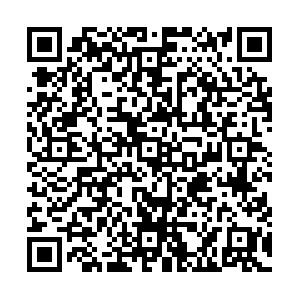# Renormalizability of leading order covariant chiral nucleon-nucleon interaction

• In this work, we study the renormalization group invariance of the recently proposed covariant power counting in the case of nucleon-nucleon scattering [Chin. Phys. C 42 (2018) 014103] at leading order. We show that unlike the Weinberg scheme, renormalizaion group invariance is satisfied in the $^3P_{0}$channel. Another interesting feature is that the $^1S_{0}$and $^3P_{1}$channels are correlated. Fixing the relevant low energy constants by fitting to the $^1S_{0}$phase shifts at $T_\mathrm{lab.}=10$and 25 MeV with cutoff values $\Lambda = 400-650$MeV, one can describe the $^3P_{1}$phase shifts relatively well. In the limit of $\Lambda\rightarrow \infty$, the $^1S_0$phase shifts become cutoff-independent, whereas the $^3P_{1}$phase shifts do not. This is consistent with the Wigner bound and previous observations that the $^{3}P_1$channel is best treated perturbatively. As for the $^1P_{1}$and $^3S_{1}$-$^3D_{1}$channels, renormalization group invariance is satisfied.
••Get Citation
Chun-Xuan Wang, Li-Sheng Geng and Bingwei Long. Renormalizability of leading order covariant chiral nucleon-nucleon interaction[J]. Chinese Physics C. doi: 10.1088/1674-1137/abe368
Chun-Xuan Wang, Li-Sheng Geng and Bingwei Long. Renormalizability of leading order covariant chiral nucleon-nucleon interaction[J]. Chinese Physics C.Milestone
Received: 2021-01-10
Article Metric

Article Views(92)
PDF Downloads(4)
Cited by(0)
Policy on re-use
To reuse of subscription content published by CPC, the users need to request permission from CPC, unless the content was published under an Open Access license which automatically permits that type of reuse.
###### 通讯作者: 陈斌, bchen63@163.com
• 1.

沈阳化工大学材料科学与工程学院 沈阳 110142

Title:
Email:

## Renormalizability of leading order covariant chiral nucleon-nucleon interaction

• 1. School of Physics, Beihang University, Beijing 102206, China
• 2. Beijing Key Laboratory of Advanced Nuclear Materials and Physics, Beihang University, Beijing 100191, China
• 3. Beijing Advanced Innovation Center for Big Data-based Precision Medicine, Beihang University, Beijing 100191, China
• 4. School of Physics and Microelectronics, Zhengzhou University, Zhengzhou 450001, China
• 5. College of Physics, Sichuan University, Sichuan 610065, China

Abstract: In this work, we study the renormalization group invariance of the recently proposed covariant power counting in the case of nucleon-nucleon scattering [Chin. Phys. C 42 (2018) 014103] at leading order. We show that unlike the Weinberg scheme, renormalizaion group invariance is satisfied in the $^3P_{0}$channel. Another interesting feature is that the $^1S_{0}$and $^3P_{1}$channels are correlated. Fixing the relevant low energy constants by fitting to the $^1S_{0}$phase shifts at $T_\mathrm{lab.}=10$and 25 MeV with cutoff values $\Lambda = 400-650$MeV, one can describe the $^3P_{1}$phase shifts relatively well. In the limit of $\Lambda\rightarrow \infty$, the $^1S_0$phase shifts become cutoff-independent, whereas the $^3P_{1}$phase shifts do not. This is consistent with the Wigner bound and previous observations that the $^{3}P_1$channel is best treated perturbatively. As for the $^1P_{1}$and $^3S_{1}$-$^3D_{1}$channels, renormalization group invariance is satisfied.

### HTMLII.   THEORETICAL FRAMEWORKIII.   RESULTS AND DISCUSSIONAPPENDIX A
Reference (59)
PDF查看关注分享

Top

### 目录/DownLoad:  Full-Size Img  PowerPoint